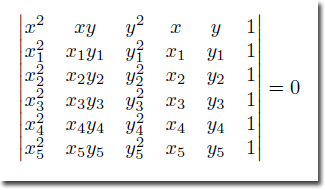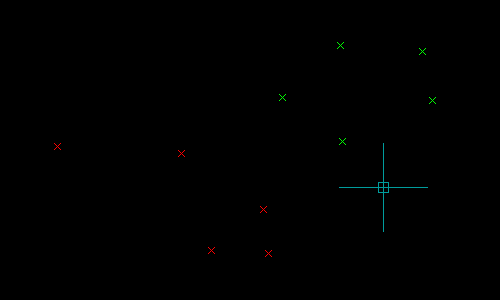# 5-Point Ellipse

This function returns the parameters defining the unique ellipse passing through five given points, if such an ellipse exists.

 Function Syntax (LM:5P-Ellipse ) Current Version 1.1 Download 5PointEllipseV1-1.lsp View HTML Version 5PointEllipseV1-1.html Donate
Arguments
Symbol Type Description
p1-5 List UCS points defining an Ellipse
Returns
Type Description
List List of:
(
(10 <WCS Center>)
(11 <WCS Major Axis Endpoint from Center>)
(40 . <Minor/Major Ratio>)
)

## Introduction

Five points are required to define a unique ellipse. Why five? Because an ellipse has five degrees of freedom: the x & y coordinates of each focus, and the sum of the distance from each focus to a point on the ellipse; (or alternatively, the x & y coordinates of the center, the length of each radius, and the rotation of the axes about the center).

The ellipse is a conic section and hence may be defined by the conic equation:Here, the values of the coefficients A, B, C, D, E & F determine whether the conic equation represents a circle, parabola, hyperbola or ellipse.

With five points, we can write a system of five conic equations, one for every supplied point, and this system may then be solved to determine the values of the above coefficients.

My function expresses this system of equations in matrix form, and determines the values of the conic equation coefficients by solving the following determinant equation:Upon calculating the values of the coefficients of the conic equation, if such values result in an ellipse, my function will proceed to derive the center, major axis endpoint relative to the center, and minor/major axis length ratio of the defined ellipse. The methods used to derive these parameters are noted in the code comments.

## Test Program

### DemonstrationThe following test program will prompt the user to pick five points and will construct an Ellipse passing through the specified points. The program demonstrates how to call the LM:5P-Ellipse function with the required parameters, and how to utilise the list returned by this function.

The program should also perform correctly in all UCS & Views.

Select all
```(defun c:test ( / i l p )
(setq i 1)
(while (and (<= i 5) (setq p (getpoint (strcat "\nSpecify Point " (itoa i) ": "))))
(setq i (1+ i)
l (cons p l)
)
)
(if (= i 6)
(entmake
(append
'(
(000 . "ELLIPSE")
(100 . "AcDbEntity")
(100 . "AcDbEllipse")
)
(apply 'LM:5P-Ellipse l)
(list (cons 210 (trans '(0.0 0.0 1.0) 1 0 t)))
)
)
)
(princ)
)
```

### Example Function Call

```_\$ (LM:5P-Ellipse '(1.0 9.0) '(1.0 5.0) '(5.0 9.0) '(7.0 8.0) '(7.0 4.0))
(
(10 4.0 6.5 0.0)
(11 -4.33735 1.15184 0.0)
(40 . 0.597178)
)
```

textsize